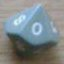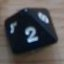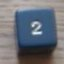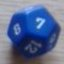How to swap dice in Pools?

## How to swap dice in Pools?

 Thu Jan 4 17:34:00 2018   by   Doug Hi, Great program however I have become stuck on trying to swap dice between 2 collections.I have dice mechanic where two players roll a pool of dice and the looser takes X hits/damage. Players roll their pool of allotted dice with the target number to equal of beat being the highest rolled number of their opponents pool. Players compare their number of successes and the loser is "awarded" the difference. In the case of players having an equal number of successes, then the player with the highest series of dice wins and awards 1 point to the loser. (Starting with the highest dice, players discard matching dice until 1 player has a higher dice.)I have managed to model the above and all works fine. What I am trying to now do is test a mechanic of: if Player A lowest dice is lower then Player B highest dice, then swap those dice. I am completely stuck and been looking at it for over a day now. Any help would be very gratefully received. Below is the simple mechanic without the dice swapping part\duelling dice pools where only the loser takes damage\opposing dice pools with tie break mechanic of first unique highest dice winsa:=5d6;b:=5d6;result := (count (max b) <= a)-(count (max a) <= b);aa := sum(max(a -- b));bb := sum(max(b -- a));tiebreak := if aa > bb then 1      else if aa < bb then -1      else 0; if result = 0  then tiebreak  else result\I had to sum the max value of a--b as it wasn't classed as a singleton? Thu Jan 4 18:33:25 2018   by   Torben First, a--b is multiset difference between a and b, and that can be empty or consist of multiple dice.  Taking the maximum of an empty set will, however, yield an empty set, so you have to sum the result to avoid empty sets when comparing.  Your program looks fine as shown for the simple case.Player A's lowest die is very likely to be smaller than Player B's highest die, so swapping is a huge advantage for player A.  Did you mean to swap when Player A's lowest die is higher than Player B's highest die?To implement swapping as you described it, you could do something like:a := 5d6;b := 5d6;if (min a) < (max b) then (  ab := (a -- (min a)) U (max b);  ba := (b -- (max b)) U (min a);  result := (count (max ba) <= ab)-(count (max ab) <= ba);  aa := sum(max(ab -- ba));  bb := sum(max(ba -- ab));  tiebreak := if aa > bb then 1      else if aa < bb then -1      else 0;  if result = 0  then tiebreak  else result) else (  result := (count (max b) <= a)-(count (max a) <= b);  aa := sum(max(a -- b));  bb := sum(max(b -- a));  tiebreak := if aa > bb then 1      else if aa < bb then -1      else 0; if result = 0  then tiebreak  else result) Fri Jan 5 10:13:02 2018   by   Doug That is brilliant, thank you!I wanted a way to see how to balance a various sized pools of Players dice, vs a pools of NPC dice. I needed to get a feel for how powerful the nuclear option would actual be. In the system I am putting together different equipment will give different powers to effect the roll. A shield may force NPC to reroll highest dice, Daggers allow players reroll lowest, Great weapon always wins tiebreakers, Parry/Repost weapon allow swap highest dice. Magic weapon, swap players lowest with NPC highest. Armour would lower opponents pool , Better weapons would increase players. In my mind this is simple mechanic that resolves a fight quickly but I need to know how to balance it all so your program is a real god send. Splendid work. Fri Jan 5 13:00:20 2018   by   Doug So expanding on a theme, is there away to pick dice type from a collection?trying to implement a reroll, keep new value but I don't know how to identify the dice type I have selected. see below:\ Opposing Dice Pools - A Rerolls Lowest\ Duelling dice pools where only the loser takes damage\ Player A rerolls thier lowest dice if they are losing.\ Tiebreak mechanic of first unique highest dice in series wins\ Doug  - 05/01/18a:=5d6;  \ Player A's poolb:=5d6;  \ Player B's poolrerollDie := d6; \ not sure how to pick dice type from a collection\ **calc winner with no reroll**result := (count (max b) <= a)-(count (max a) <= b);aa := sum(max(a -- b));bb := sum(max(b -- a));tiebreak := if aa > bb then 1      else if aa < bb then -1      else 0;result := if result = 0  then tiebreak  else result;\ **If A has lost then reroll**if result < 0 then (  ar:= (a -- (min a)) U (rerollDie);   resultR := (count (max b) <= ar)-(count (max ar) <= b);aa := sum(max(ar -- b));bb := sum(max(b -- ar));tiebreak := if aa > bb then 1      else if aa < bb then -1      else 0;if resultR = 0 then tiebreak  else resultR)else result Fri Jan 5 15:13:28 2018   by   Torben I'm not sure what you mean by picking a dice type from a collection.  If you mean the lowest or highest, that can be done with min or max, as you do above.  For, say, the second lowest from a, you can use `max (least 2 a)`.Or did you mean something else? Mon Jan 8 09:21:54 2018   by   Doug If I havea := d4 U d6 U d12and I want to reroll min a